Magic Square
 What is a Magic 3x3 Square?  The Magic 3x3 Square The Magic 4x4 Square The Magic 5x5 Square The Magic nxn Square Curiosities Simple Variants Magic Squares on the Internet References ..
 To the Main Page       "Mathematische Basteleien"

What is a Magic 3x3 Square?
 ..............8....1....6......................... ..............3....5....7......................... ..............4....9....2......................... You can assemble the numbers 1 to 9 in a square, so that the sum of the rows, the columns, and the diagonals is 15. If you take the numbers 1 to 9, you have the standard square.

 .............8+c....1+c....6+c.............. .............3+c....5+c....7+c.............. .............4+c....9+c....2+c.............. A magic square remains magic, if you change each numbers by a constant c. You add c on the left. You can also subtract, multiply or divide.
You define 4x4-, 5x5-... squares correspondingly.

The Magic 3x3 Square top
You have 1+2+3+4+5+6+7+8+9=45. In a magic square you have to add 3 numbers again and again. Therefore the average sum of three numbers is 45:3=15. The number 15 is called the magic number of the 3x3 square.
You can also achieve 15, if you add the middle number 5 three times.

You can reduce 15 in a sum of three summands eight times:
 15=1+5+9  15=1+6+8 15=2+4+9 15=2+5+8 15=2+6+7  15=3+4+8 15=3+5+7  15=4+5+6
The odd numbers 1,3,7, and 9 occur twice in the reductions, the even numbers 2,4,6,8 three times and the number 5 once.
Therefore you have to place number 5 in the middle of the magic 3x3 square. The remaining odd numbers have to be in the middles of a side and the even numbers at the corners.
Under these circumstances there are eight possibilities building a square: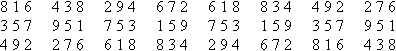All the eight squares change into each other, if you reflect them at the axes of symmetry. You count symmetric squares only once. Therefore there is only one magic 3x3 square.

The Magic 4x4 Squaretop
The magic number is (1+2+...+15+16):4 = 34.
The computer found 86 reductions of 34 to a sum of four summands with the numbers 1 to 16.
 34=01+02+15+16  34=01+03+14+16 34=01+04+13+16  34=01+04+14+15  34=01+05+12+16  34=01+05+13+15  34=01+06+11+16  34=01+06+12+15  34=01+06+13+14  34=01+07+10+16  34=01+07+11+15  34=01+07+12+14 34=01+08+09+16  34=01+08+10+15  34=01+08+11+14  34=01+08+12+13  34=01+09+10+14  34=01+09+11+13 34=01+10+11+12  34=02+03+13+16  34=02+03+14+15  34=02+04+12+16  34=02+04+13+15  34=02+05+11+16 34=02+05+12+15  34=02+05+13+14  34=02+06+10+16  34=02+06+11+15 34=02+06+12+14  34=02+07+09+16 34=02+07+10+15  34=02+07+11+14  34=02+07+12+13  34=02+08+09+15  34=02+08+10+14  34=02+08+11+13 34=02+09+10+13 34=02+09+11+12  34=03+04+11+16  34=03+04+12+15  34=03+04+13+14  34=03+05+10+16  34=03+05+11+15  34=03+05+12+14  34=03+06+09+16  34=03+06+10+15  34=03+06+11+14  34=03+06+12+13 34=03+07+08+16  34=03+07+09+15  34=03+07+10+14  34=03+07+11+13  34=03+08+09+14  34=03+08+10+13 34=03+08+11+12  34=03+09+10+12  34=04+05+09+16  34=04+05+10+15  34=04+05+11+14  34=04+05+12+13  34=04+06+08+16  34=04+06+09+15  34=04+06+10+14  34=04+06+11+13  34=04+07+08+15  34=04+07+09+14 34=04+07+10+13  34=04+07+11+12  34=04+08+09+13  34=04+08+10+12  34=04+09+10+11  34=05+06+07+16 34=05+06+08+15  34=05+06+09+14  34=05+06+10+13 34=05+06+11+12  34=05+07+08+14  34=05+07+09+13 34=05+07+10+12  34=05+08+09+12  34=05+08+10+11  34=06+07+08+13  34=06+07+09+12  34=06+07+10+11 34=06+08+09+11  34=07+08+09+10  . . . .
The summands 1 to 16 are distributed regularly in the reductions:
 Summand: Number: 01 19 02 20 03 21 04 22 05 22 06 23 07 23 08 22 09 22 10 23 11 23 12 22 13 22 14 21 15 20 16 19
Unlike the 3x3 square there is not just one conclusion for the distribution of the numbers 1 to 16 in a 4x4 square.

Fact: There are 880 magic squares, counting the symmetric ones only once.
This is one of  880 possible squares:
 ............12....06....15.....01............ ............13....03....10.....08............ ............02....16....05.....11............ ............07....09....04.....14............ This square is special. The number 34 is not only the sum of the numbers in the rows, the columns, and both diagonals, but also in every 2x2 square.

The Magic 5x5 Square top
The magic number is (1+2+...+24+25) : 5 = 65
Reductions of the magic number  65.
 65 = 01+02+13+24+25 65 = 01+02+14+23+25 65 =  ... 65 =  ... 65 =  ... 65 =  ... 65 = 10+12+13+14+16 65 = 11+12+13+14+15 The computer found 1394 reductions of the number 65.
The summands and their number in the sums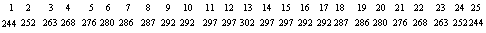You notice, that the middle number 13 = 65:5 appears most frequently. The numbers of the summands to smaller and bigger summands drop symmetrically to both sides.

Fact: There are 275 305 224 magic 5x5 squares. (Scientific American 1/1976)

Making of a magic 5x5-square:
You go through the numbers 1 to 25. There are two rules for constructing a magic square "top right" and "if the place is occupied, go one down".
 .....................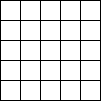.................... Number 1 is placed in the centre of the first row.  Number 2 follows top right. But then you leave the 5x5 square.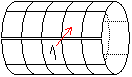Therefore you must imagine the square is a cylinder. The cylinder has the vertical square sides of the square as circumference. The horizontal sides touch each other and close the curved surface of the cylinder. So there is a field top right for number 2. If you unroll the cylinder, number 2 has gone to the last row one place to the right.
Number 3 follows top right.
 ...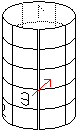... Number 4 would lie outside the 5x5 square. So again you imagine the square would become a cylinder, this time with vertical axis. You can find a place for number 4. If you unroll the cylinder, you find the number in the third row on the far left.
Number 5 lies top right.
You use the second rule for number 6. If the field top right is occupied, you put the number one row down in the same column.
You go further on with 7, 8, and so on.  - You use the same rule for number 16 as for number 6.

You can transfer this way of  formation to all magic squares with odd numbers of the sides ;-).
There are also rules for magic squares with an even side length. They are more complicated however.

The Magic nxn Squareetop
The existence of magic squares nxn is proved for all numbers n>2. But there is no general rule.
The magic number is (1 + 2 + 3 + ... + n²) : n =0.5 * (n²+1) * n.

The magic numbers of the standard squares:
Magic squares:    3x3    4x4     5x5     6x6     7x7      8x8      9x9     10x10
Magic numbers:   15      34      65      111     175      260      369      505

Curiosities   top
 ...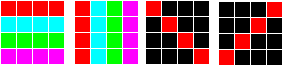Once again: A square is magic, if the numbers have the same sum in the rows, the columns and the diagonals.

 ...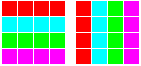... A square is semi-magic, if the numbers have the same sum only in the rows and the columns.

 ...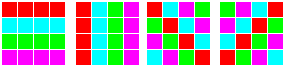... A square is pandiagonal, if it is magic and if not only the numbers of the main diagonals, but also the broken diagonals have the same sum.
 ...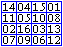This magic square is pandiagonal.

 ...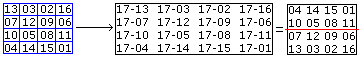... You find the complementary square, if you replace each number n by 17-n.
The square is self-complemetary, because the new one is symmetric to the old one. See red axis.

 ...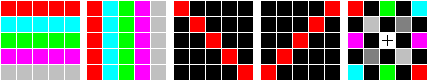... A square is associative, if it is magic and if pairs of numbers lying symmetrically to the centre have the same sum. The sum is 26=5²+1 =n²+1.
 ...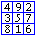The 3x3 magic square Lo Shu is associative.

You can fix the kind of the numbers.
 ...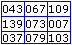A magic square with prime numbers

 ...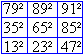A "semi"-magic square of square numbers All sums going through the centre have the same value.  Source: http://www.mathpages.com/home/kmath417.htm

The sequence of these special magic squares is not finished. There are magic squares missing e.g.
>panmagic squares
>magic squares formed by smaller magic squares
>bordered magic squares (Book 2).

There is an explanation for all the different magic squares: They can be found by computers. A new property means a new query inside the program.

Simple Variants top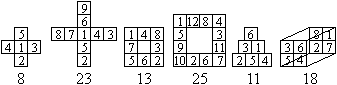Magic Squares on the Internet     top

English

Craig Knecht (Magic Square Models)
 Water Retention Patterns ...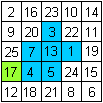... Imagine, the squares are the top of square prisms with the height given by the numbers. If you pour water in this solid, it stays in the centre upto the height 17. Then it flows off. The amount of water is (17-3)+(17-7)+(17-13)+(17-1)+(17-4)+(17-5)=69. There are nice problems: Biggest amount of water? Seperate ponds? Island?

Eric W. Weisstein (MathWorld)
Magic Square, Panmagic Square, Associative Magic SquareLo Shu

Harvey D. Heinz
Magic Squares, Magic Stars & Other Patterns

Holger Danielsson
Magic Squares

Ivars Peterson's MathTrek
More than Magic Squares

Mark S. Farrar
Magic Squares

MathPages
Solving Magic Squares

Robin Moseley
Magic Flexagon   (.pdf-File)

German

Feng-Shui-Homepage

Gerd Müller

Hans-Peter Gramatke

Holger Danielsson

Jan Theofel und Martin Trautmann

Maria Koth

Paul Heimbach

recordholders.org
Das größte Magische Quadrat der Welt

Udo Hebisch  (Mathematisches Café)

References  top
(1) Bild der Wissenschaften, Heft 8/1966, Heft 6/1968, Heft 10/1976
(2) Pieter van Delft /Jack Botermans: Denkspiele der Welt, München 1980 (1998 neu aufgelegt)
(3) Maximilian Miller, Gelöste und ungelöste mathematische Probleme, Leipzig 1982

Feedback: Email address on my main page